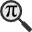## General

Display information for equation id:math.219960.8 on revision:219960

* Page found: Kurtosis (eq math.219960.8)

(force rerendering)

Cannot find the equation data in the database. Fetching from revision text.

Occurrences on the following pages:

Hash: a97118fb9e8d7e006a466bfc0771f888

TeX (original user input):

X_i


TeX (checked):

X_{i}


### LaTeXML (experimental; uses MathML) rendering

MathML (843 B / 337 B) :$X_{i}$
<math xmlns="http://www.w3.org/1998/Math/MathML" id="p1.1.m1.1" class="ltx_Math" alttext="{\displaystyle X_{i}}" display="inline">
<semantics id="p1.1.m1.1a">
<msub id="p1.1.m1.1.3" xref="p1.1.m1.1.3.cmml">
<mi id="p1.1.m1.1.1" xref="p1.1.m1.1.1.cmml">X</mi>
<mi id="p1.1.m1.1.2.1" xref="p1.1.m1.1.2.1.cmml">i</mi>
</msub>
<annotation-xml encoding="MathML-Content" id="p1.1.m1.1b">
<apply id="p1.1.m1.1.3.cmml" xref="p1.1.m1.1.3">
<csymbol cd="ambiguous" id="p1.1.m1.1.3.1.cmml" xref="p1.1.m1.1.3">subscript</csymbol>
<ci id="p1.1.m1.1.1.cmml" xref="p1.1.m1.1.1">𝑋</ci>
<ci id="p1.1.m1.1.2.1.cmml" xref="p1.1.m1.1.2.1">𝑖</ci>
</apply>
</annotation-xml>
<annotation encoding="application/x-tex" id="p1.1.m1.1c">{\displaystyle X_{i}}</annotation>
</semantics>
[/itex]


SVG (2.33 KB / 1.205 KB) :

### MathML with SVG or PNG fallback (recommended for modern browsers and accessibility tools) rendering

SVG image empty. Force Re-Rendering

SVG (0 B / 8 B) :

PNG (0 B / 8 B) :

$X_{i}$## Translations to Computer Algebra Systems

### Translation to Maple

In Maple: X[i]

I: You use a typical letter for a constant [the imaginary unit == the principal square root of -1].

We keep it like it is! But you should know that Maple uses I for this constant.

If you want to translate it as a constant, use the corresponding DLMF macro \iunit

i: the imaginary unit == the principal square root of -1 was translated to: i

### Translation to Mathematica

In Mathematica: Subscript[X, i]

I: You use a typical letter for a constant [the imaginary unit == the principal square root of -1].

We keep it like it is! But you should know that Mathematica uses I for this constant.

If you want to translate it as a constant, use the corresponding DLMF macro \iunit

i: the imaginary unit == the principal square root of -1 was translated to: i

## Similar pages

Calculated based on the variables occurring on the entire Kurtosis page

## Identifiers

• $X_{i}$### MathML observations

0results

0results

no statistics present please run the maintenance script ExtractFeatures.php

0 results

0 results